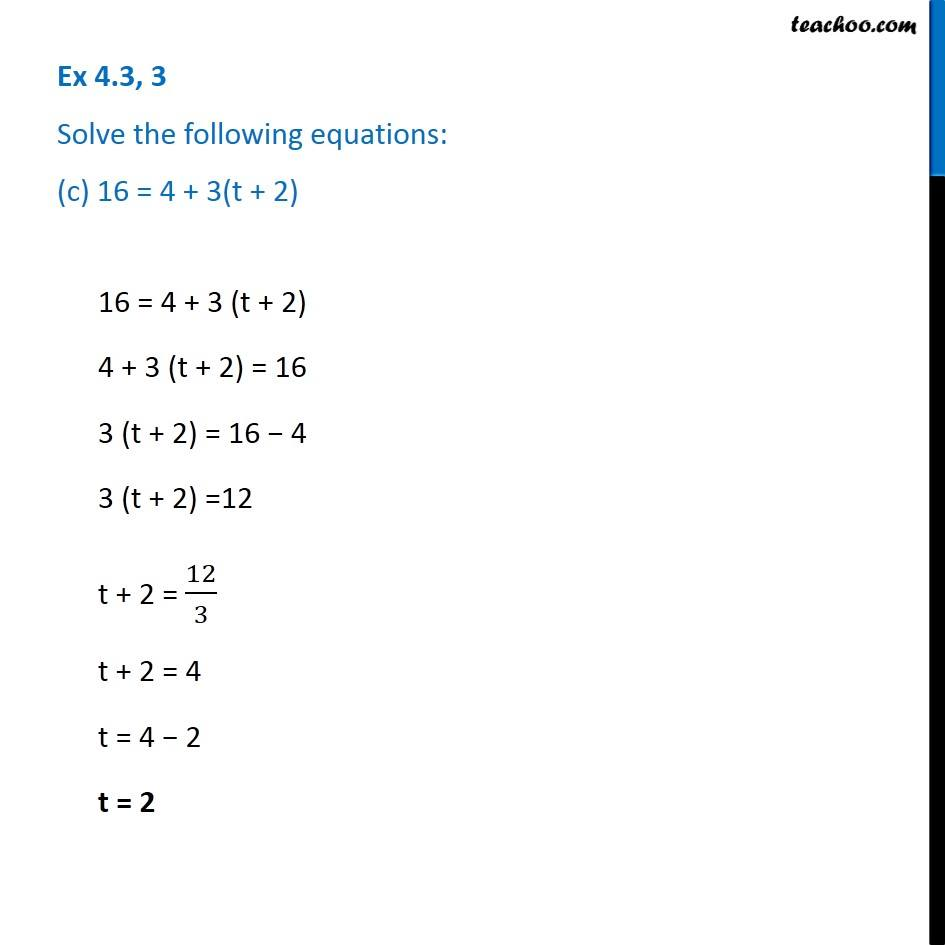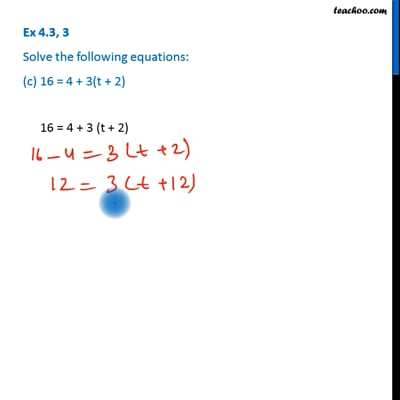Ex 4.3

Chapter 4 Class 7 Simple Equations
Serial order wiseThis video is only available for Teachoo black users

Get live Maths 1-on-1 Classs - Class 6 to 12

### Transcript

Ex 4.3, 3 Solve the following equations: (c) 16 = 4 + 3(t + 2)16 = 4 + 3 (t + 2) 4 + 3 (t + 2) = 16 3 (t + 2) = 16 − 4 3 (t + 2) =12 t + 2 = 12/3 t + 2 = 4 t = 4 − 2 t = 2